# Examples: Perfect Fractional Exponents

In math, we often learn one set of facts through calculation, and another through related calculations. For example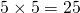. We can calculate this one. The related fact is that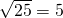. We know this fact through association rather than calculation. Later, when solving algebraic equations involving a square, we will also need to remember that.

Or how about this,. The associated number fact is that,. We don’t ‘calculate’ that the number multiplied by itself 5 times over to make 32 is 2: we know it from memory.

To help with remembering exponent facts, here is a short list of most commonly used powers: Exponent Cheat Sheet. If you don’t already recall these facts easily, keep the reference sheet handy so that you can focus on the concepts rather than the arithmetic. Here is a long list from wikipedia.

# Evaluating positive fractional exponents

Generally, a fractional exponent leads to an irrational number. These questions are called ‘perfect’ because the answers are all integers, and can be evaluated without using a calculator.

Example1:We’re asking, what power of four is equal to 16? This is the part that we recall. (For the fourth root you can also do this little trick – square root then square root):Or just check the Exponent Cheat Sheet.

Example 2First, we need to know that. Without knowing that, this question is simply really hard.

This is how we handle it:

We know that.

And we know thatThis is why we can rewriteasIn this form, we first evaluate the bracket from memory then calculate the power:Example 3:We mean, take the cube root of 125 then square it.Example 4We mean, take the square root of 64 then cube.Example 5:Now,isn’t on the cheat sheet. So let’s look for a likely relative. We find: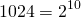Now,And,Therefore,.

Now we have it,.

# Evaluating negative exponents

Division can be expressed usingor by using a fraction or by using a negative exponent. A negative exponent does not make the value negative because dividing does not make a value negative.

1 divided by 7 can be written as:Note that all of these are positive (all are equal to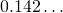). Generally the first thing we do with a negative exponent (which means ‘divide’) is to write it as a fraction (another way to say ‘divide’).

Example 6:A negative exponent represents repeated division. When we seein algebra, we understand that it means, but theis not necessary to write. Here, it can be helpful to write the 1, as seen in the second line: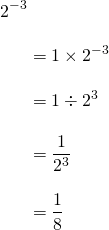Example 7:# Fractions with Negative Exponents

In examples 8, 9 and 10 we take a negative power of a fraction. To understand the process, you need to remember how to divide a fraction. Here’s a reminder: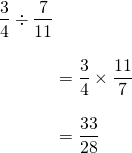Example 8:Watch what happens to the fraction when there is a negative power.Notice that our orignial fraction flips when the exponent is negative. Let’s write that down in general:

In general,Example 9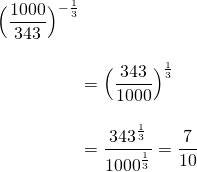Example 10Top